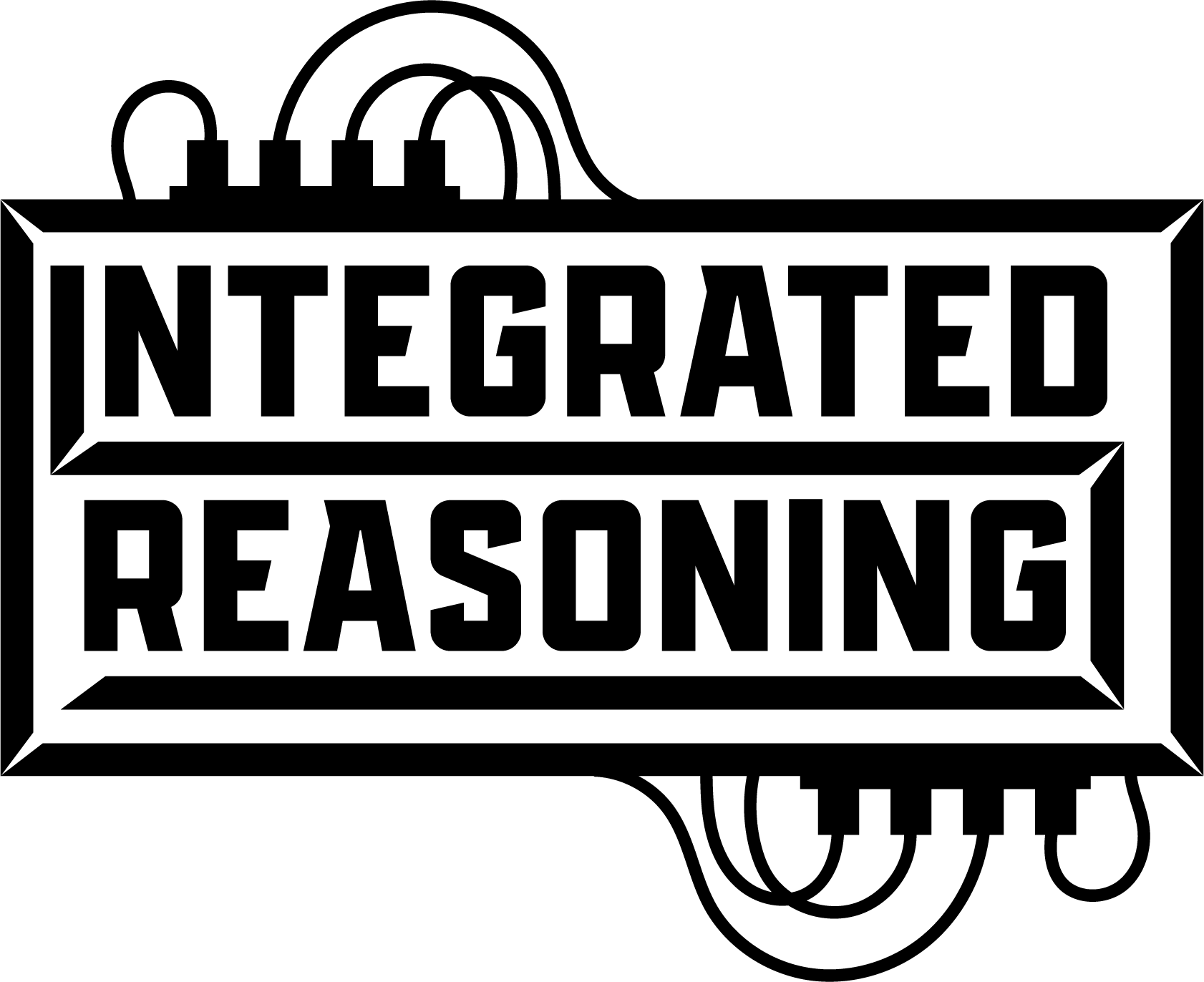# Subset Sum in Python

Quickly calculate answers to the Subset Sum Problem

This API solves the decision formulation of the subset sum problem for all positive integers. You can find out more about the subset sum problem on the wikipedia page. Using our API sends the data over to our servers running our specialized hardware, where your problem is quickly solved and then the solution is returned back. You can interact through our web API, or use our Python APIs in order to interact with our hardware.

# Python Interface

## Input and Output Format

Inputs are specified as a python list. This list represents the set that you would like to run subset sum over. Order does not matter in this list.

Input Limitations: We currently support at least 1 and up to 120 positive integers in the input. The maximum integer supported is 2^31 - 1 and the total sum cannot exceed 17,180,000,000.

Output the standard binary output is a list of 64-bit integers. Bit X of the entry at index Y is the answer for whether a subset of the input can sum to (64*Y+X). So, if the result has one entry which is 0x5 (0b101), then we know that the possible sums are 0 and 2. In order to determine if there's a subset that sums to 131, we'd check bit 3 of entry 2, because (64 * 2 + 3) is 131.

## API Usage

### subset_sum_result_check(binary, index)

This method is used to check what the standard binary output, as defined in Input and Output Format has encoded for the item at index.

So, if you want to know if a subset of [5, 7, 60] sums to 12, you would first pass that set to one of the complete subset sum methods specified below. Then you would pass the binary output of that method to this method with index '12', and this method will return True, because the subset [5, 7] sums to 12.

Arg binary is a standard output binary as specified in Input and Output Format.

Arg index is the number to check in the binary.

Returns: The output will be True if the binary does include index, and will be False if it does not.

Behavior: This API will block until the operation completes. This will happen locally and should be reasonably quick, O(n) where n is the length of the binary input.

### complete_subset_sum(input_list)

This will synchronously solve the complete subset sum for the input.

Arg input_list is the input list as specified in Input and Output Format.

Returns: The output will be the standard binary output as specified in Input and Output Format. Will throw an exception when there is an error.

Behavior: This API will block until the operation completes. This may take some time, as this will cover networking time, queued time, and the time it takes to solve the problem.

Example code:

``````import irx

input_set = [5, 7, 60]
result = irx.complete_subset_sum(input_set)

print("The output for input set [5, 7, 60] is ", result, result)

does_sum_to_12 = "Yes" if irx.subset_sum_result_check(result, 12) else "No"

print("Does a subset of [5, 7, 60] sum to 12? ", does_sum_to_12)

does_sum_to_70 = "Yes" if irx.subset_sum_result_check(result, 1) else "No"

print("Does a subset of [5, 7, 60] sum to 70? ", does_sum_to_70)

``````

### complete_subset_sum_async(input_list, callback)

This will asynchronously solve the complete subset sum for the input.

Arg input_list is the input list as specified in Input and Output Format.

Arg input_list is the callback function to be called upon completion. Callback must take exactly one argument, which will be the result binary as specified in Input and Output Format

Returns: does not return anything. Will throw an exception when there is an error.

Behavior: This API will return immediately. There may be some time before the callback is called, as this will be after networking time, queued time, and the time it takes to solve the problem.

Example code:

``````import irx

wait_sem = Semaphore(0)

input_set = [5, 7, 60]

def check_output(binary_result):
print("The output for input set [5, 7, 60] is ", binary_result, binary_result)
does_sum_to_12 = "Yes" if irx.subset_sum_result_check(binary_result, 12) else "No"
print("Does a subset of [5, 7, 60] sum to 12? ", does_sum_to_12)
does_sum_to_70 = "Yes" if irx.subset_sum_result_check(binary_result, 1) else "No"
print("Does a subset of [5, 7, 60] sum to 70? ", does_sum_to_70)
wait_sem.release()

irx.complete_subset_sum_async(input_set, check_output)
wait_sem.acquire()
``````

# See another example

There's a full demo at Subset Sum Demo that you can check out to see this in action.